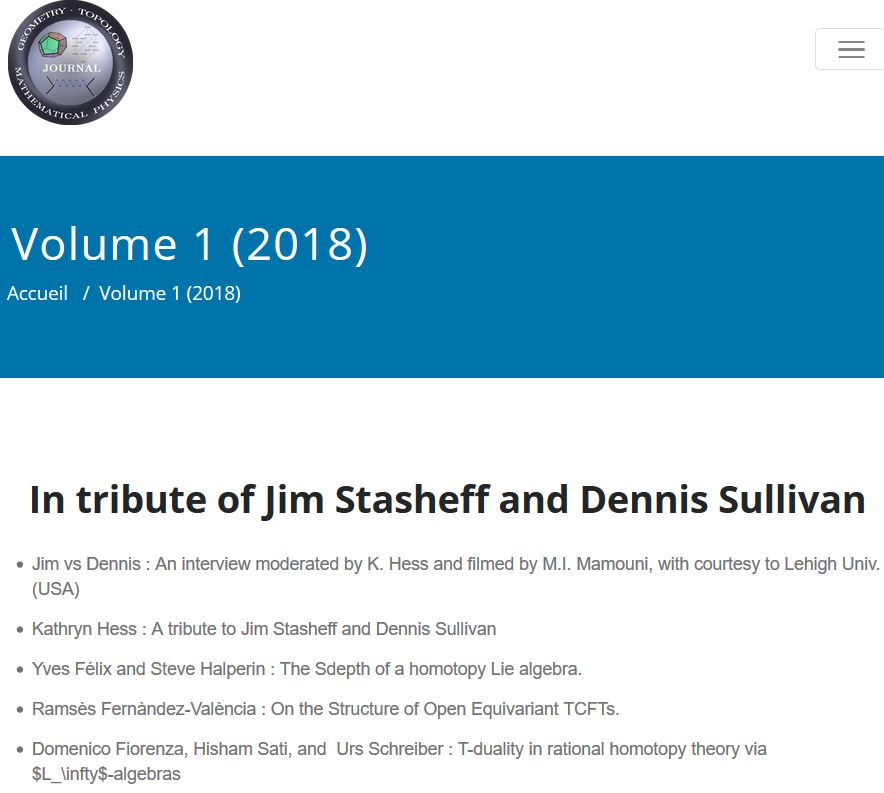# Schreiber T-duality in rational homotopy theory via strong homomotopy Lie algebras

An article that we have written:• T-duality in rational homotopy theory via $L_\infty$-algebras

GTMP, Vol 1 (2018)

on rational topological T-duality in superstring theory formulated via L-∞ algebras.

Abstract We combine Sullivan models from rational homotopy theory with Stasheff‘s L-∞ algebras to describe a duality in string theory. Namely, what in string theory is known as topological T-duality between K0-cocycles in type IIA string theory and K1-cocycles in type IIB string theory, or as Hori’s formula, may be recognized as a Fourier-Mukai transform between twisted cohomologies when regarded through the lenses of rational homotopy theory. We show this as an example of topological T-duality in rational homotopy theory, which in turn can be completely formulated in terms of morphisms of L-∞ algebras.

$\,$

This is a review, making the Fourier-Mukai transform manifest, of

Talk slides:

Lecture notes:

This is based on our previous articles:

Last revised on August 9, 2020 at 07:34:27. See the history of this page for a list of all contributions to it.